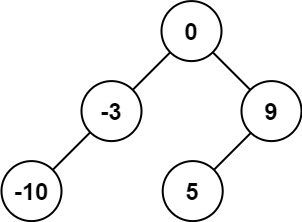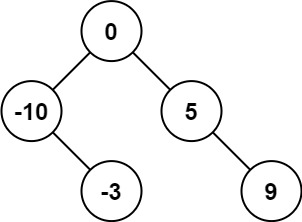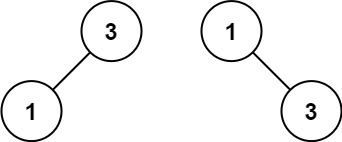# 108. Convert Sorted Array to Binary Search Tree

https://leetcode.com/problems/convert-sorted-array-to-binary-search-tree/

Given an integer array nums where the elements are sorted in ascending order, convert it to a height-balanced binary search tree. A height-balanced binary tree is a binary tree in which the depth of the two subtrees of every node never differs by more than one.

Example 1:Input: nums = [-10,-3,0,5,9] Output: [0,-3,9,-10,null,5] Explanation: [0,-10,5,null,-3,null,9] is also accepted:Example 2:Input: nums = [1,3] Output: [3,1] Explanation: [1,null,3] and [3,1] are both height-balanced BSTs.

Constraints:

``````1 <= nums.length <= 104
-104 <= nums[i] <= 104
nums is sorted in a **strictly increasing** order.
``````

• code
``````class Solution {
public TreeNode sortedArrayToBST(int[] nums) {
if (nums.length == 0) return null;
int mid = nums.length / 2;
TreeNode root = new TreeNode(nums[mid]);
root.left = sortedArrayToBST(Arrays.copyOf(nums, mid));
root.right = sortedArrayToBST(Arrays.copyOfRange(nums, mid + 1, nums.length));
return root;
}
}
``````
• c mid = (len(nums)-1)//2 also works
``````class Solution:

def sortedArrayToBST(self, nums: List[int]) -> TreeNode:
if not nums:
return None

mid = len(nums)//2